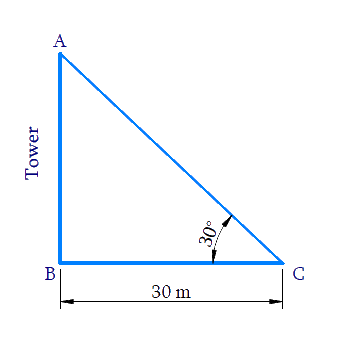# Ex.9.1 Q4 Some Applications of Trigonometry Solution - NCERT Maths Class 10

Go back to  'Ex.9.1'

## Question

The angle of elevation of the top of a tower from a point on the ground, which is $$30\,\rm{m}$$ away from the foot of the tower, is $$30^\circ$$. Find the height of the tower.

Video Solution
Some Applications Of Trigonometry
Ex 9.1 | Question 4

## Text Solution

What is Known?

(i) Angle of elevation of the top of the tower from a point on ground is $$30^\circ$$

(ii) Distance between the foot of the tower to the point on the ground is $$30\,\rm{m.}$$

What is Unknown?

Height of the tower,Reasoning:

Let us consider the height of the tower as $$AB,$$ distance between the foot of tower to the point on ground as $$BC .$$

In $$\Delta ABC$$,

Trigonometric ratio involving $$AB, BC$$ and $$\angle C$$ is $$\tan \theta$$.

Steps:

In $$\Delta ABC$$,

\begin{align}\tan \,C &= \frac{{AB}}{{BC}}\\\tan 30^\circ &= \frac{{AB}}{{30}}\\\frac{1}{{\sqrt 3 }} &= \frac{{AB}}{{30}}\\AB &= \frac{{30}}{{\sqrt 3 }}\\&= \frac{{30}}{{\sqrt 3 }} \times \frac{{\sqrt 3 }}{{\sqrt 3 }}\\ &= \frac{{30\sqrt 3 }}{3}\\&= 10\sqrt 3 \end{align}

Height of tower $$AB$$ $$=10 \sqrt{3} \mathrm{m}$$

Learn from the best math teachers and top your exams

• Live one on one classroom and doubt clearing
• Practice worksheets in and after class for conceptual clarity
• Personalized curriculum to keep up with school